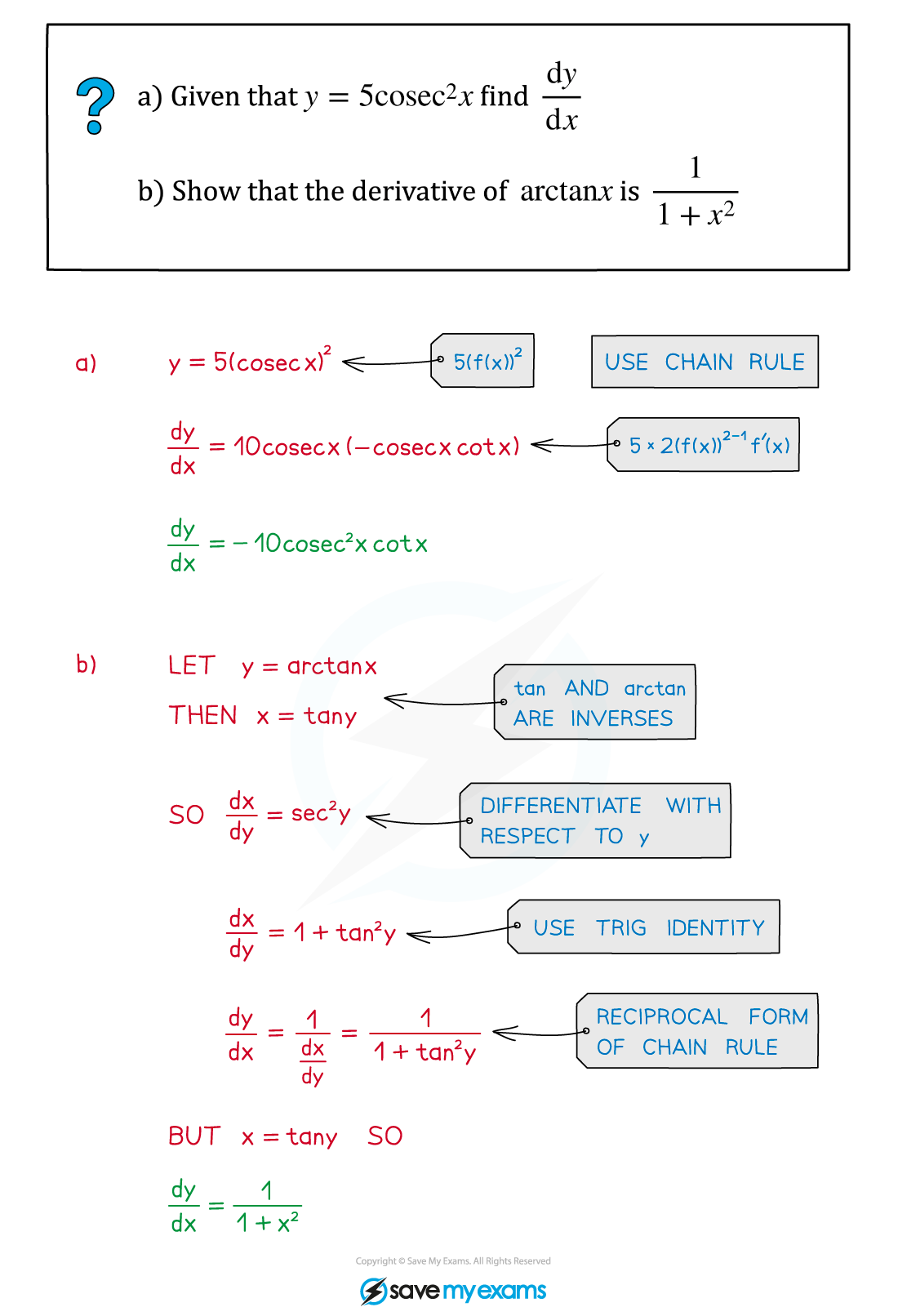# AQA A Level Maths: Pure复习笔记7.3.6 Differentiating Reciprocal and Inverse Trig Functions

### Differentiating Reciprocal and Inverse Trig Functions

#### How do I differentiate the reciprocal trigonometric functions?

• The formulae for the derivatives of the reciprocal trigonometric functions are: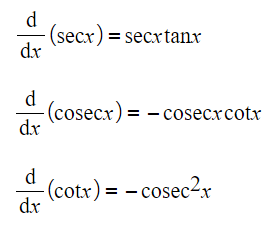• You can derive the derivatives for sec, cosec, and cot using the chain rule and the derivatives of the basic trigonometric functions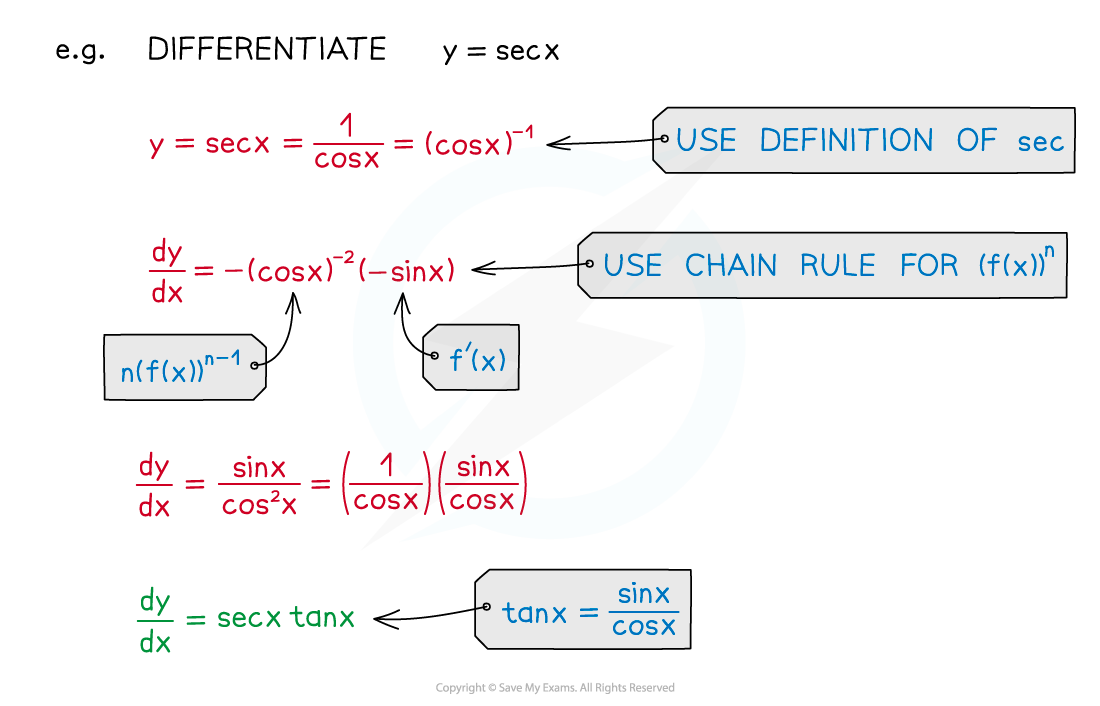#### How do I differentiate the inverse trigonometric functions?

• The formulae for the derivatives of the inverse trigonometric functions are: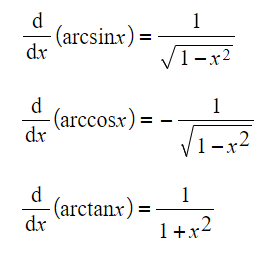• You can derive the derivatives of arcsin, arccos, and arctan using the reciprocal form of the chain rule and the derivatives of the basic trigonometric functions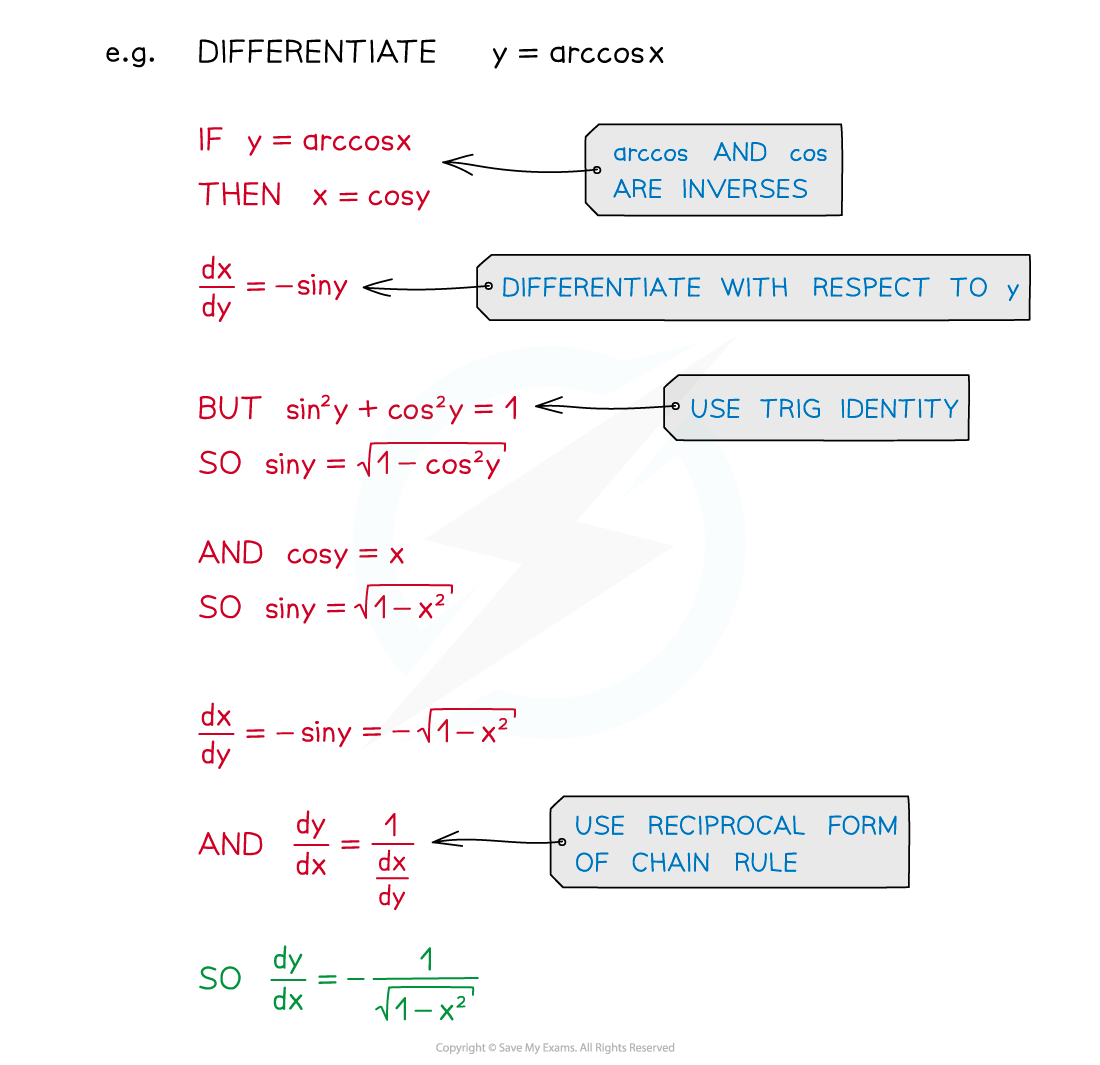#### Exam Tip

• The formulae for the derivatives of sec x, cosec x, and cot x are in the  formulae booklet – you don't need to memorise them
• However, you should know how to derive the derivatives of sec, cosec, and cot using the chain rule
• The formulae for the derivatives of arcsin, arccos, and arctan are not in the formulae booklet
• You should know how to derive those derivatives using the reciprocal form of the chain rule

#### Worked Example